# 图像处理中的点检测

## 图像处理--角点检测

2015-11-02 19:44:46 samkieth 阅读数 13683
• ###### 数字图像处理实战

本课程内容主要选取自stanford EE368/CS...本次课程包含图像算法和机器学习，编程工具主要包括python，c++，android，matlab，opencv，Tesseract等。 由于个人时间精力有限，课程将每隔2-3周发布一次。 </p>

7课时 65分钟 680人学习 金圣韬
免费试看

# 角点及角点检测

①角点是一阶导数的局部最大值所对应的像素点；
②角点是指两条以上的边缘的交点；
③角点指示了物体边缘变化不连续的方向；
④角点处的一阶导数最大，而且高阶导数为零；
⑤角点处不仅梯度的数值大，而且梯度方向的变化率也很大。也就是说，角点指示

(角点也是边缘点的一部分)，又考虑了梯度方向的变化率(只有当梯度方向剧烈变化时才

# 角点检测算法

## 1.FAST算法

### 1.1定义

Rosten等人将FAST角点定义为：若某像素点与其周围领域内足够多的像素点处于不同的区域，则该像素点可能为角点。也就是某些属性与众不同，考虑灰度图像，即若该点的灰度值比其周围领域内足够多的像素点的灰度值大或者小，则该点可能为角点。### 1.2fast算法实现步骤

1. 从图片中选取一个像素\$P\$，下面我们将判断它是否是一个特征点。我们首先把它的亮度值设为\$I_p\$。
2. 设定一个合适的阈值\$t\$。
3. 考虑以该像素点为中心的一个半径等于3像素的离散化的Bresenham圆，这个圆的边界上有16个像素（如图1所示）。
4. 现在，如果在这个大小为16个像素的圆上有\$n\$个连续的像素点，它们的像素值要么都比\$I_p + t\$大，要么都比\$I_p - t\$小，那么它就是一个角点。（如图1中的白色虚线所示）。\$n\$的值可以设置为12或者9，实验证明选择9可能会有更好的效果。

1. 当\$n<12\$时不能拒绝许多的候选点；
2. 检测出来的角点不是最优的，这是因为它的效率取决于问题的排序与角点的分布；
3. 对于角点分析的结果被丢弃了；
4. 多个特征点容易挤在一起。

### 1.3采用非极大值抑制

1. 为每一个检测到的特征点计算它的响应大小（score function）\$V\$。这里\$V\$定义为点\$p\$和它周围16个像素点的绝对偏差的和。
2. 考虑两个相邻的特征点，并比较它们的\$V\$值。
3. \$V\$值较低的点将会被删除。

### 1.4fast算法在opencv实现

```void FAST(InputArray image,vector<KeyPoint>& keypoints,int threshold,boolnonmaxSuppression=true )

C++:void FASTX(InputArray image,vector<KeyPoint>& keypoints,int threshold,boolnonmaxSuppression, int type)```

OpenCV里对FAST的使用也非常简单，先声明一组特征点，构建FAST特征检测，接下来调用detect函数检测图像中的特征点，最后把特征点绘制到图片上。上代码说的清楚些。
```#include <opencv2/core/core.hpp>
#include <opencv2/features2d/features2d.hpp>
#include <opencv2/highgui/highgui.hpp>

#include <vector>

using namespace cv;

void main()
{
Mat image;
// vector of keyPoints
std::vector<KeyPoint> keyPoints;
// construction of the fast feature detector object
FastFeatureDetector fast(40);   // 检测的阈值为40
// feature point detection
fast.detect(image,keyPoints);
drawKeypoints(image, keyPoints, image, Scalar::all(255), DrawMatchesFlags::DRAW_OVER_OUTIMG);
imshow("FAST feature", image);
cvWaitKey(0);
} ```### 1.5fast算法总结

FAST算法比其他已知的角点检测算法要快很多倍，但是当图片中的噪点较多时，它的健壮性并不好，而且算法的效果还依赖于一个阈值\$t\$。

## 图像处理之霍夫变换（直线检测算法）

2012-07-07 14:42:21 jia20003 阅读数 109368
• ###### 数字图像处理实战

本课程内容主要选取自stanford EE368/CS...本次课程包含图像算法和机器学习，编程工具主要包括python，c++，android，matlab，opencv，Tesseract等。 由于个人时间精力有限，课程将每隔2-3周发布一次。 </p>

7课时 65分钟 680人学习 金圣韬
免费试看

X *cos(theta) + y * sin(theta)  = r 其中角度theta指r与X轴之间的夹角，r为到直线几何垂(x –a ) ^2 + (y-b) ^ 2 = r^2其中(a, b)为圆的中心点坐标，r圆的半径。这样霍夫的参数空间就

1.      读取一幅带处理二值图像，最好背景为黑色。

2.      取得源像素数据

3.      根据直线的霍夫变换公式完成霍夫变换，预览霍夫空间结果

4.       寻找最大霍夫值，设置阈值，反变换到图像RGB值空间(程序难点之一)

5.      越界处理，显示霍夫变换处理以后的图像

``` // prepare for hough transform
int centerX = width / 2;
int centerY = height / 2;
double hough_interval = PI_VALUE/(double)hough_space;

int max = Math.max(width, height);
int max_length = (int)(Math.sqrt(2.0D) * max);
hough_1d = new int[2 * hough_space * max_length];```

```// start hough transform now....
int[][] image_2d = convert1Dto2D(inPixels);
for (int row = 0; row < height; row++) {
for (int col = 0; col < width; col++) {
int p = image_2d[row][col] & 0xff;
if(p == 0) continue; // which means background color

// since we does not know the theta angle and r value,
// we have to calculate all hough space for each pixel point
// then we got the max possible theta and r pair.
// r = x * cos(theta) + y * sin(theta)
for(int cell=0; cell < hough_space; cell++ ) {
max = (int)((col - centerX) * Math.cos(cell * hough_interval) + (row - centerY) * Math.sin(cell * hough_interval));
max += max_length; // start from zero, not (-max_length)
if (max < 0 || (max >= 2 * max_length)) {// make sure r did not out of scope[0, 2*max_lenght]
continue;
}
hough_2d[cell][max] +=1;
}
}
}```

```// find the max hough value
int max_hough = 0;
for(int i=0; i<hough_space; i++) {
for(int j=0; j<2*max_length; j++) {
hough_1d[(i + j * hough_space)] = hough_2d[i][j];
if(hough_2d[i][j] > max_hough) {
max_hough = hough_2d[i][j];
}
}
}
System.out.println("MAX HOUGH VALUE = " + max_hough);

// transfer back to image pixels space from hough parameter space
int hough_threshold = (int)(threshold * max_hough);```

```	    // transfer back to image pixels space from hough parameter space
int hough_threshold = (int)(threshold * max_hough);
for(int row = 0; row < hough_space; row++) {
for(int col = 0; col < 2*max_length; col++) {
if(hough_2d[row][col] < hough_threshold) // discard it
continue;
int hough_value = hough_2d[row][col];
boolean isLine = true;
for(int i=-1; i<2; i++) {
for(int j=-1; j<2; j++) {
if(i != 0 || j != 0) {
int yf = row + i;
int xf = col + j;
if(xf < 0) continue;
if(xf < 2*max_length) {
if (yf < 0) {
yf += hough_space;
}
if (yf >= hough_space) {
yf -= hough_space;
}
if(hough_2d[yf][xf] <= hough_value) {
continue;
}
isLine = false;
break;
}
}
}
}
if(!isLine) continue;

// transform back to pixel data now...
double dy = Math.sin(row * hough_interval);
double dx = Math.cos(row * hough_interval);
if ((row <= hough_space / 4) || (row >= 3 * hough_space / 4)) {
for (int subrow = 0; subrow < height; ++subrow) {
int subcol = (int)((col - max_length - ((subrow - centerY) * dy)) / dx) + centerX;
if ((subcol < width) && (subcol >= 0)) {
image_2d[subrow][subcol] = -16776961;
}
}
} else {
for (int subcol = 0; subcol < width; ++subcol) {
int subrow = (int)((col - max_length - ((subcol - centerX) * dx)) / dy) + centerY;
if ((subrow < height) && (subrow >= 0)) {
image_2d[subrow][subcol] = -16776961;
}
}
}
}
}``````package com.gloomyfish.image.transform;

import java.awt.image.BufferedImage;

import com.process.blur.study.AbstractBufferedImageOp;

public class HoughLineFilter extends AbstractBufferedImageOp {
public final static double PI_VALUE = Math.PI;
private int hough_space = 500;
private int[] hough_1d;
private int[][] hough_2d;
private int width;
private int height;

private float threshold;
private float scale;
private float offset;

public HoughLineFilter() {
// default hough transform parameters
//	scale = 1.0f;
//	offset = 0.0f;
threshold = 0.5f;
scale = 1.0f;
offset = 0.0f;
}

public void setHoughSpace(int space) {
this.hough_space = space;
}

public float getThreshold() {
return threshold;
}

public void setThreshold(float threshold) {
this.threshold = threshold;
}

public float getScale() {
return scale;
}

public void setScale(float scale) {
this.scale = scale;
}

public float getOffset() {
return offset;
}

public void setOffset(float offset) {
this.offset = offset;
}

@Override
public BufferedImage filter(BufferedImage src, BufferedImage dest) {
width = src.getWidth();
height = src.getHeight();

if ( dest == null )
dest = createCompatibleDestImage( src, null );

int[] inPixels = new int[width*height];
int[] outPixels = new int[width*height];
getRGB( src, 0, 0, width, height, inPixels );
houghTransform(inPixels, outPixels);
setRGB( dest, 0, 0, width, height, outPixels );
return dest;
}

private void houghTransform(int[] inPixels, int[] outPixels) {
// prepare for hough transform
int centerX = width / 2;
int centerY = height / 2;
double hough_interval = PI_VALUE/(double)hough_space;

int max = Math.max(width, height);
int max_length = (int)(Math.sqrt(2.0D) * max);
hough_1d = new int[2 * hough_space * max_length];

// define temp hough 2D array and initialize the hough 2D
hough_2d = new int[hough_space][2*max_length];
for(int i=0; i<hough_space; i++) {
for(int j=0; j<2*max_length; j++) {
hough_2d[i][j] = 0;
}
}

// start hough transform now....
int[][] image_2d = convert1Dto2D(inPixels);
for (int row = 0; row < height; row++) {
for (int col = 0; col < width; col++) {
int p = image_2d[row][col] & 0xff;
if(p == 0) continue; // which means background color

// since we does not know the theta angle and r value,
// we have to calculate all hough space for each pixel point
// then we got the max possible theta and r pair.
// r = x * cos(theta) + y * sin(theta)
for(int cell=0; cell < hough_space; cell++ ) {
max = (int)((col - centerX) * Math.cos(cell * hough_interval) + (row - centerY) * Math.sin(cell * hough_interval));
max += max_length; // start from zero, not (-max_length)
if (max < 0 || (max >= 2 * max_length)) {// make sure r did not out of scope[0, 2*max_lenght]
continue;
}
hough_2d[cell][max] +=1;
}
}
}

// find the max hough value
int max_hough = 0;
for(int i=0; i<hough_space; i++) {
for(int j=0; j<2*max_length; j++) {
hough_1d[(i + j * hough_space)] = hough_2d[i][j];
if(hough_2d[i][j] > max_hough) {
max_hough = hough_2d[i][j];
}
}
}
System.out.println("MAX HOUGH VALUE = " + max_hough);

// transfer back to image pixels space from hough parameter space
int hough_threshold = (int)(threshold * max_hough);
for(int row = 0; row < hough_space; row++) {
for(int col = 0; col < 2*max_length; col++) {
if(hough_2d[row][col] < hough_threshold) // discard it
continue;
int hough_value = hough_2d[row][col];
boolean isLine = true;
for(int i=-1; i<2; i++) {
for(int j=-1; j<2; j++) {
if(i != 0 || j != 0) {
int yf = row + i;
int xf = col + j;
if(xf < 0) continue;
if(xf < 2*max_length) {
if (yf < 0) {
yf += hough_space;
}
if (yf >= hough_space) {
yf -= hough_space;
}
if(hough_2d[yf][xf] <= hough_value) {
continue;
}
isLine = false;
break;
}
}
}
}
if(!isLine) continue;

// transform back to pixel data now...
double dy = Math.sin(row * hough_interval);
double dx = Math.cos(row * hough_interval);
if ((row <= hough_space / 4) || (row >= 3 * hough_space / 4)) {
for (int subrow = 0; subrow < height; ++subrow) {
int subcol = (int)((col - max_length - ((subrow - centerY) * dy)) / dx) + centerX;
if ((subcol < width) && (subcol >= 0)) {
image_2d[subrow][subcol] = -16776961;
}
}
} else {
for (int subcol = 0; subcol < width; ++subcol) {
int subrow = (int)((col - max_length - ((subcol - centerX) * dx)) / dy) + centerY;
if ((subrow < height) && (subrow >= 0)) {
image_2d[subrow][subcol] = -16776961;
}
}
}
}
}

// convert to hough 1D and return result
for (int i = 0; i < this.hough_1d.length; i++)
{
int value = clamp((int)(scale * this.hough_1d[i] + offset)); // scale always equals 1
this.hough_1d[i] = (0xFF000000 | value + (value << 16) + (value << 8));
}

// convert to image 1D and return
for (int row = 0; row < height; row++) {
for (int col = 0; col < width; col++) {
outPixels[(col + row * width)] = image_2d[row][col];
}
}
}

public BufferedImage getHoughImage() {
BufferedImage houghImage = new BufferedImage(hough_2d.length, hough_space, BufferedImage.TYPE_4BYTE_ABGR);
setRGB(houghImage, 0, 0, hough_2d.length, hough_space, hough_1d);
return houghImage;
}

public static int clamp(int value) {
if (value < 0)
value = 0;
else if (value > 255) {
value = 255;
}
return value;
}

private int[][] convert1Dto2D(int[] pixels) {
int[][] image_2d = new int[height][width];
int index = 0;
for(int row = 0; row < height; row++) {
for(int col = 0; col < width; col++) {
index = row * width + col;
image_2d[row][col] = pixels[index];
}
}
return image_2d;
}

}
```

## 图像处理之角点检测与亚像素角点定位

2016-12-14 11:31:09 jia20003 阅读数 11536
• ###### 数字图像处理实战

本课程内容主要选取自stanford EE368/CS...本次课程包含图像算法和机器学习，编程工具主要包括python，c++，android，matlab，opencv，Tesseract等。 由于个人时间精力有限，课程将每隔2-3周发布一次。 </p>

7课时 65分钟 680人学习 金圣韬
免费试看

1. 基于灰度图像、能够自动调整运行稳定，检测出角点的数目。

2. 对噪声不敏感、有一定的噪声抑制，有较强的角点角点检测能力。

3. 准确性够高，能够正确发现角点位置

4. 算法尽可能的快与运行时间短

Harris角点检测基本上满足了上述四点要求，所以被广发应用，除了Harris角点检测，另外一种常见的角点检测算法-Shi-Tomasi角点检测也得到了广发应用，OpenCV中对这两种算法均有实现API可以调用。关于Harris角点检测原理可以看我之前写的博文：

http://blog.csdn.net/jia20003/article/details/169086611. 基于插值方法

2. 基于几何矩寻找方法

3. 拟合方法 - 比较常用OpenCV亚像素角点检测例子：

```#include <opencv2/opencv.hpp>
#include <iostream>

using namespace cv;
using namespace std;

Mat src, gray_src;
int max_corners = 10;
int max_trackbar = 30;
const char* output_title = "subpxiel-result";
void GoodFeature2Track_Demo(int, void*);
int main(int argc, char** argv) {
if (src.empty()) {
return -1;
}
cvtColor(src, gray_src, COLOR_BGR2GRAY);
namedWindow("input", CV_WINDOW_AUTOSIZE);
namedWindow(output_title, CV_WINDOW_AUTOSIZE);
imshow("input", src);

createTrackbar("Corners:", output_title, &max_corners, max_trackbar, GoodFeature2Track_Demo);
GoodFeature2Track_Demo(0, 0);

waitKey(0);
return 0;
}

void GoodFeature2Track_Demo(int, void*) {
if (max_corners < 1) {
max_corners = 1;
}
vector<Point2f> corners;
double qualityLevel = 0.01;
double minDistance = 10;
int blockSize = 3;
double k = 0.04;
goodFeaturesToTrack(gray_src, corners, max_corners, qualityLevel, minDistance, Mat(), blockSize, false, k);
cout << "number of corners : " << corners.size() << endl;
Mat copy = src.clone();
for (size_t t = 0; t < corners.size(); t++) {
circle(copy, corners[t], 4, Scalar(255, 0, 0), 2, 8, 0);
}
imshow(output_title, copy);

// locate corner point on sub pixel level
Size winSize = Size(5, 5);
Size zerozone = Size(-1, -1);
TermCriteria criteria = TermCriteria(TermCriteria::EPS + TermCriteria::MAX_ITER, 40, 0.001);
cornerSubPix(gray_src, corners, winSize, zerozone, criteria);
for (size_t t = 0; t < corners.size(); t++) {
cout << (t+1) << ".point[x, y]=" << corners[t].x << "," << corners[t].y << endl;
}

return;
}```## 图像处理中经典的特征检测和提取算法

2019-09-10 10:23:03 u014410989 阅读数 497
• ###### 数字图像处理实战

本课程内容主要选取自stanford EE368/CS...本次课程包含图像算法和机器学习，编程工具主要包括python，c++，android，matlab，opencv，Tesseract等。 由于个人时间精力有限，课程将每隔2-3周发布一次。 </p>

7课时 65分钟 680人学习 金圣韬
免费试看

### 图像处理中经典的特征检测和提取算法

OpenCV中最常使用的特征检测和提取算法有：
Harris、SIFT、SURF、FAST、BRIEF、ORB等。

# 引言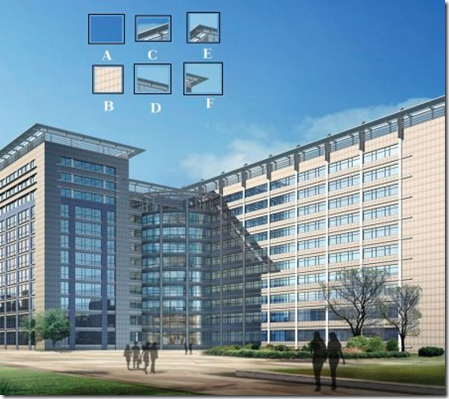E,F中的角我们通常称作角点（corner points），他们具有以下特征：

–局部窗口沿各方向移动，均产生明显变化的点

–图像局部曲率突变的点

# 1. Moravec角点检测

Moravec角点检测用一个二值窗口在图像的某个像素点的所有方向上进行移动，并计算移动后和移动前像素强度变化的平均值，得到的***最小***的值定为该点的角点响应值。用公式表示，即为：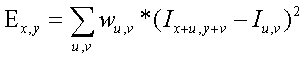x，y是待检测点的图像坐标。
u，v是待检测点邻域图像块的移动方向。
w是可调节的权重。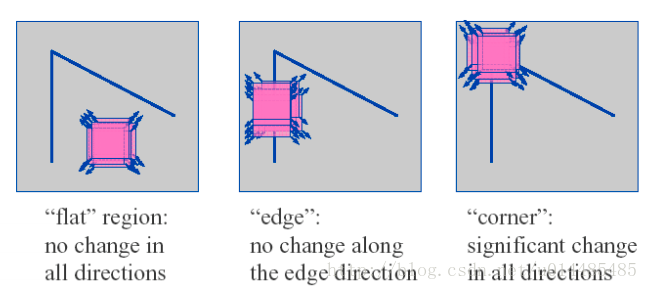一般计算这个像素点水平、垂直、对角线、反对角线4个方向上的灰度差平方和。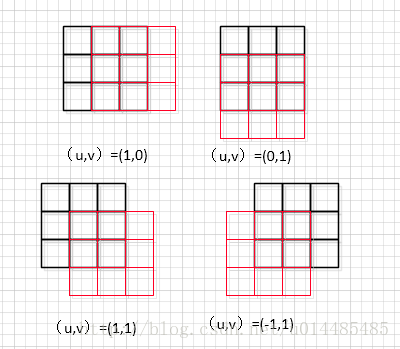# 2. Harris

1、Harris 角点检测（Harris Corner Detection）（OpenCV实现）
https://blog.csdn.net/u014485485/article/details/79056666

2、Harris Corner Detector 原理及编程实现
https://blog.csdn.net/tanhongguang1/article/details/8898927

Harris角点检测是Chris Harris和Mike Stephens在1988年提出的。主要用于运动图像的追踪。
Harris角点检测来自于Moravec检点检测（1977，Moravec），并对它进行了改进和更强的数学建模。
OpenCV 函数：cornerHarris

Moravec角点有一些缺点，导致它的检测不精确，Harris改良了这些缺点。
1.Moravec响应是各向异性的，因为只考虑了45度倍数方向上的响应。
Harris通过对shift region进行泰勒展开，覆盖所有方向的移动。
2.Moravec响应容易受到噪声的干扰，因为窗口是二值的，且为方形。
Harris改用具有平滑效果的高斯圆形窗口。
3.Moravec响应对边缘、角点的判决过于简单，因为响应只考虑E的最小值。
Harris重新设计了一种衡量边缘、角点的方式——利用E的方差。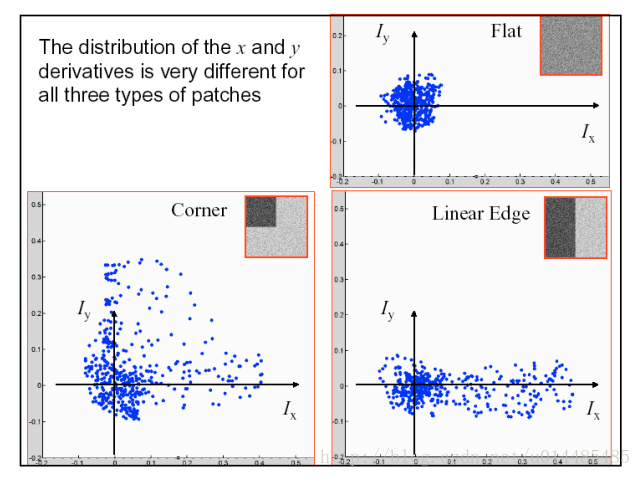Harris角点的缺点：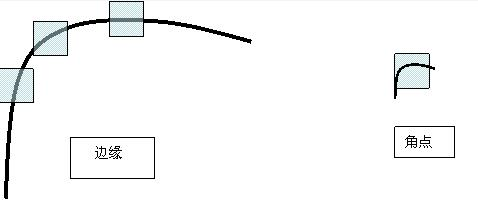# 3.SIFT

Harris角点具有平移不变形和旋转不变形，但是没有尺度不变形。
SIFT对于平移、旋转、尺度缩放、亮度变化都保持不变性。

SIFT，即尺度不变特征变换（Scale-invariant feature transform，SIFT），是用于图像处理领域的一种描述。这种描述具有尺度不变性，可在图像中检测出关键点，是一种局部特征描述子。
SIFT由David Lowe在1999年提出，在2004年加以完善 [1-2] 。SIFT在数字图像的特征描述方面当之无愧可称之为最红最火的一种，许多人对SIFT进行了改进，诞生了SIFT的一系列变种。SIFT已经申请了专利。

The SIFT algorithm is patented in the United States and cannot be used in commercial products without a license from the University of British Columbia.

``````pip install opencv-contrib-python
``````

1、David G. Lowe, “Distinctive image features from scale-invariant keypoints,” International Journal of Computer Vision, 60, 2 (2004), pp. 91-110. [PDF][CODE][project homepage][author’s homepage]

2、原理讲解（中文）
[SIFT算法详解及应用，讲解全面形象，百度文库下载]
[SIFT算法详解及应用，讲解全面形象，CSDN下载]

[SIFT算法的Matlab实现（blog） ]
zddhub, SIFT算法详解: http://blog.csdn.net/zddblog/article/details/7521424
Rachel Zhang, SIFT特征提取分析: http://blog.csdn.net/abcjennifer/article/details/7639681
JiePro, SIFT算法：特征描述子: http://www.cnblogs.com/JiePro/p/sift_4.html

3、代码

Rob Hess, OpenSIFT源码，C语言版本: https://github.com/robwhess/opensift
SIFT算法的Matlab实现（github下载）matlab语言版本 ：https://github.com/sun11/sw-sift

# 4. SURF

SIFT特征和SURF特征比较: https://blog.csdn.net/blateyang/article/details/76512398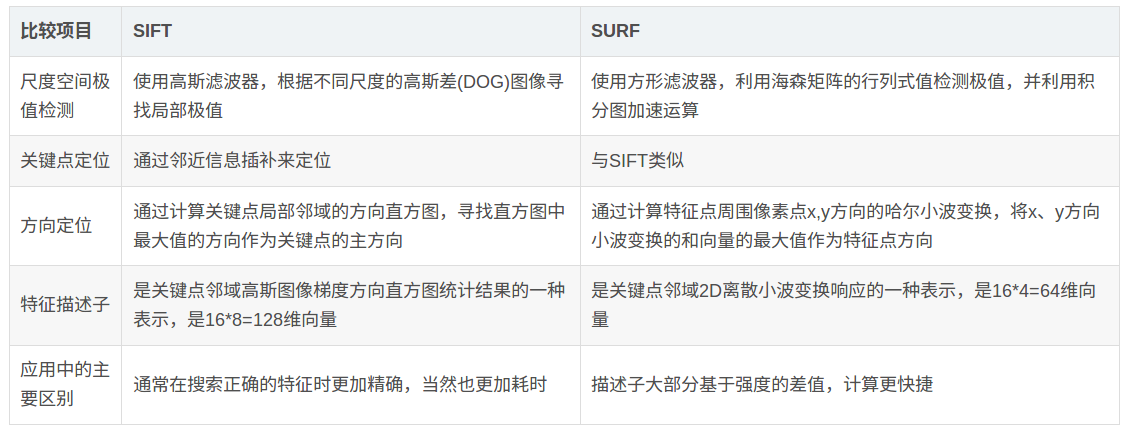# 5. FAST

FAST的全称为Features From Accelerated Segment Test。(FAST主要用于角点检测)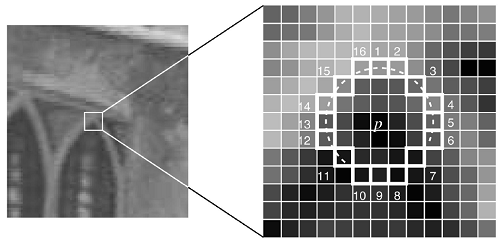1.从图片中选取一个像素P，下面我们将判断它是否是一个特征点。我们首先把它的亮度值设为Ip。
2.设定一个合适的阈值t。
3.考虑以该像素点为中心的一个半径等于3像素的离散化的Bresenham圆，这个圆的边界上有16个像素（如图1所示）。
4.现在，如果在这个大小为16个像素的圆上有n个连续的像素点，它们的像素值要么都比Ip+t大，要么都比Ip−t小，那么它就是一个角点。（如图1中的白色虚线所示）。n的值可以设置为12或者9，实验证明选择9可能会有更好的效果。

Features From Accelerated Segment Test：
https://www.cnblogs.com/ronny/p/4078710.html?utm_source=tuicool

# 6. BRIEF

EPFL的Calonder在ECCV2010上提出了一种可以快速计算且表达方式为二进制编码的描述子。

SIFT特征采用了128维的特征描述子，由于描述子用的浮点数，所以它将会占用512 bytes的空间。

BRIEF提供了一种计算二值串的捷径，而并不需要去计算一个类似于SIFT的特征描述子。它需要先平滑图像，然后在特征点周围选择一个Patch，在这个Patch内通过一种选定的方法来挑选出来nd个点对。然后对于每一个点对(p,q)，我们来比较这两个点的亮度值，如果I§>I(q)，则这个点对生成了二值串中一个的值为1，如果I§<I(q)，则对应在二值串中的值为-1，否则为0。所有nd个点对，都进行比较之间，我们就生成了一个nd长的二进制串。

SIFT、SURF、Harris、BRIEF、FAST、DAISY、FAST等描述符介绍：https://blog.csdn.net/qq_29828623/article/details/52403562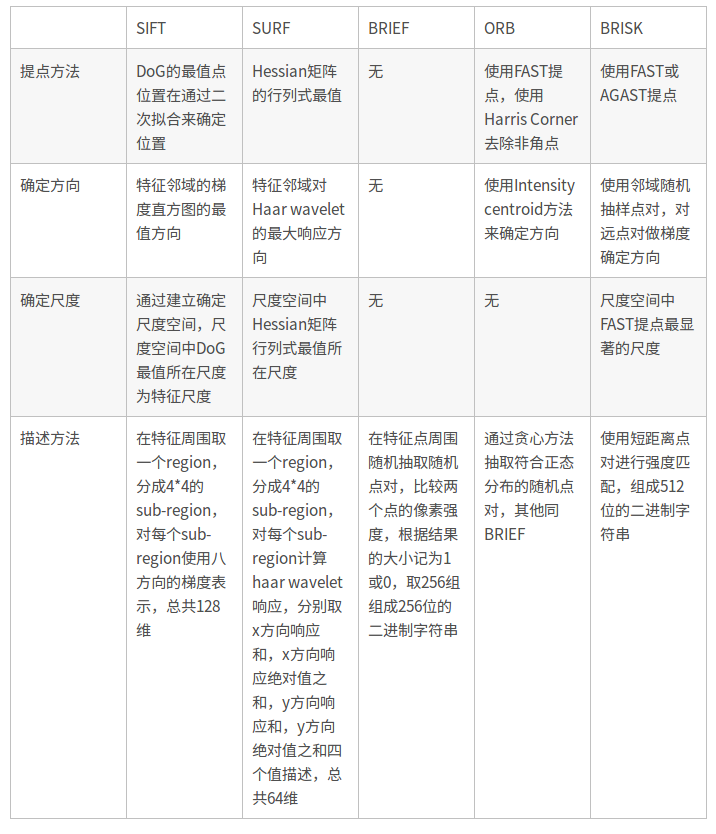# 7. ORB

ORB是Oriented FAST and Rotated BRIEF的简称。ORB将在本文中详细描述。Ethan Rublee and Vincent Rabaud and Kurt Konolige and Gary Bradski,《ORB: an efficient alternative to SIFT or SURF》, ICCV 2011。OpenCV2.3中已经实现。

1、对于FAST算法，增加了快速准确的方向指向功能；
2、高运算效率的具有指向功能的BRIEF特征；
3、具有指向功能的BRIEF特征的方差及相关性分析；
4、基于旋转不变且去关联性的BRIEF特征的方法，用于减少近邻取样（点取样）应用中。

SURF SIFT ORB三种特征检测算法比较： https://blog.csdn.net/zilanpotou182/article/details/66478915
ORB 一种特征匹配替代方法：对比SIFT或SURF ： https://blog.csdn.net/u012525173/article/details/70332181

# 8. LBP

LBP(Local Binary Pattern)，局部二值模式是一种描述图像局部纹理的特征算子，该算子是由T.Ojala等人于1994年首次提出的，后经过发展改进可应用于图像特征分析，该算子具有旋转不变性与灰度不变性(不怕光照变化)等显著优点。

LBP特征描述的是一种灰度范围内的图像处理操作技术。LBP特征是高效的图像特征分析方法，经过改进与发展已经应用于多个领域之中，特别是人脸识别、表情识别、行人检测领域已经取得了成功。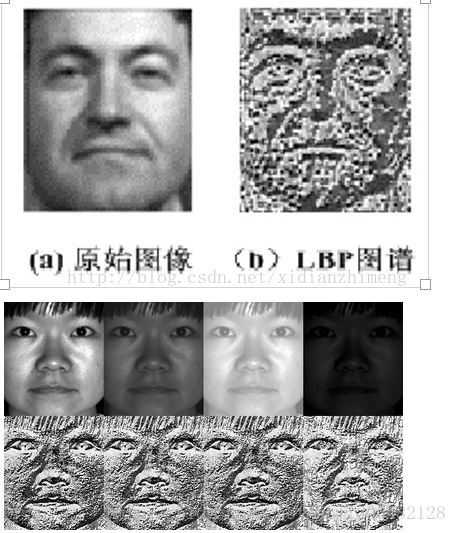# 9. HOG

HOG主要捕获轮廓信息。统计每个cell的梯度直方图，然后若干个cell直方图组成一个block的descriptor。所有block组成整幅图片的descriptor。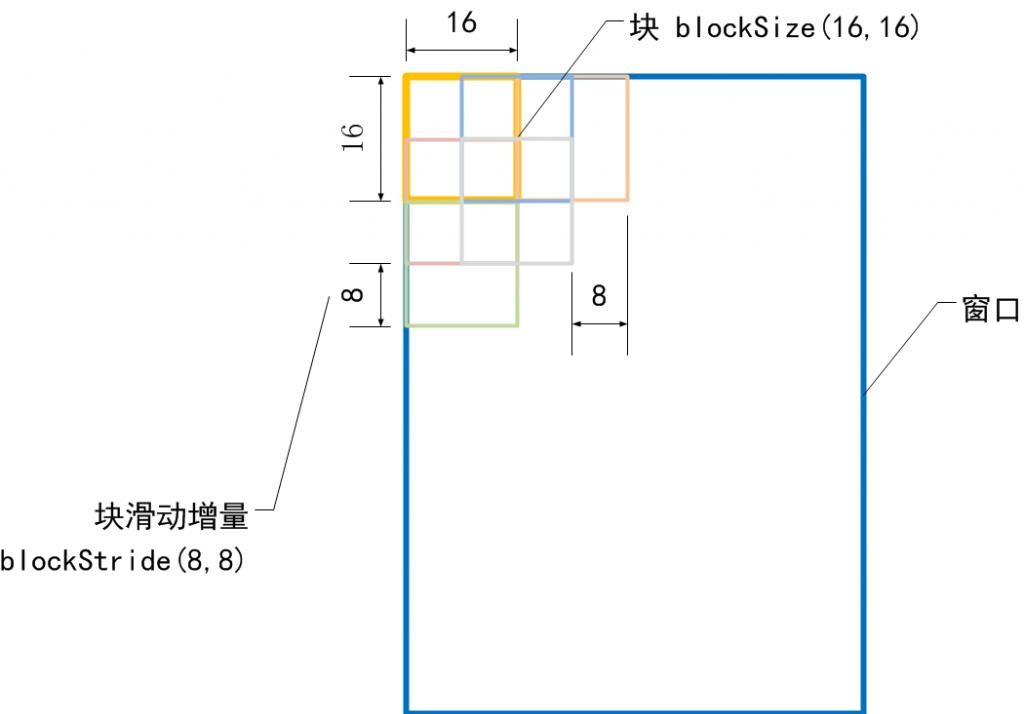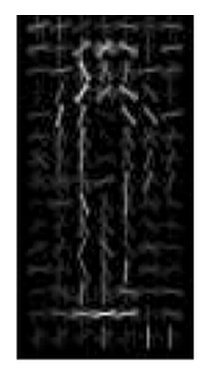# 10. HAAR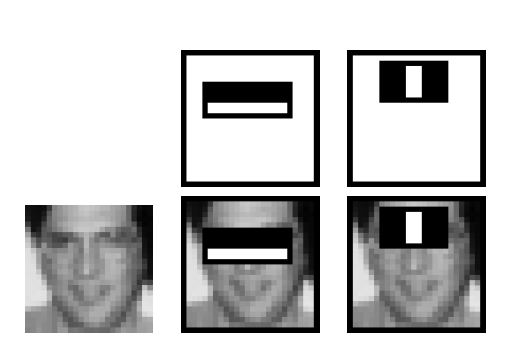• HAAR特征只是使用特征模板，计算图像颜色的深浅模式，模板以不同的大小和位置在子图像中滑动，计算出特征值来，交给boost分类器。其实HAAR与LBP还是有点像的，都是灰度差。
• HAAR计算的模式也决定它对旋转没有很好的适应性

# 11. Feature Detection Methods List:

https://blog.csdn.net/vonzhoufz/article/details/46594369

1. Canny Edge Detect,
A Computational Approach to Edge Detection, 1986. The Canny edge detector is an edge detection operator that uses a multi-stage algorithm to detect a wide range of edges in images.

2. Harris,
A combined corner and edge detector, 1988. considering the differential of the corner score with respect to direction directly.

3. GFTT，
Good Features to Track,1994, Determines strong corners on an image.

4. Matas-2000,
Robust Detection of Lines Using the Progressive Probabilistic Hough Transform. 霍夫变换检测直线.

5. SIFT,
Distinctive Image Features from Scale-Invariant Keypoints,2004, invariant to image translation, scaling, and rotation, partially invariant to illumination changes and robust to local geometric distortion. 128-dim(512B).

6. SURF,
Speeded Up Robust Features,2006,受SIFT启发，比SIFT快，健壮. 64-dim(256B).

7. FAST ,
Machine Learning for High-speed Corner Detection, 2006,wiki. Very fast, not robust to high level noise.

8. ORB,
ORB: an efficient alternative to SIFT or SURF,2011，基于FAST和BRIEF，比SIFT快两个数量级，可作为SIFT的替代(a fusion of FAST keypoint detector and BRIEF descriptor). 32B binary descriptor.

9. BRISK，
BRISK: Binary Robust Invariant Scalable Keypoints, 2011 . 64B binary descriptor.

10. STAR，
Censure: Center surround extremas for realtime feature detection and matching,2008,引用次数不高.scale-invariant center-surround detector (CENSURE) that claims to outperform other detectors and is capable of real-time implementation.

11. MSER，
Robust Wide Baseline Stereo from Maximally Stable Extremal Regions, 2002, 斑点检测(blob detection).

## 图像处理中边缘检测和轮廓检测的区别

2016-09-03 15:58:29 NNNNNNNNNNNNY 阅读数 13057
• ###### 数字图像处理实战

本课程内容主要选取自stanford EE368/CS...本次课程包含图像算法和机器学习，编程工具主要包括python，c++，android，matlab，opencv，Tesseract等。 由于个人时间精力有限，课程将每隔2-3周发布一次。 </p>

7课时 65分钟 680人学习 金圣韬
免费试看

1. http://wangmurong.org.cn/2015/10/21/edge-detection-segmentation-contour-detection/
2. Opencv官方例程中的contours2.cpp
3. 《OpenCV3编程入门》 浅墨_毛星云